# 步骤

1. 建立层次结构模型
2. 构造判断（成对比较）矩阵
3. 层次单排序及其一致性检验
4. 层次总排序及其一致性检验

## 建立层次结构模型

• 最高层：决策的目的，要解决的问题。eg.选择哪里的房子
• 最底层：决策时的备选方案。eg.不同地方的房子
• 中间层：考虑的因素、决策的准则。eg.价格、舒适度、环境、交通便利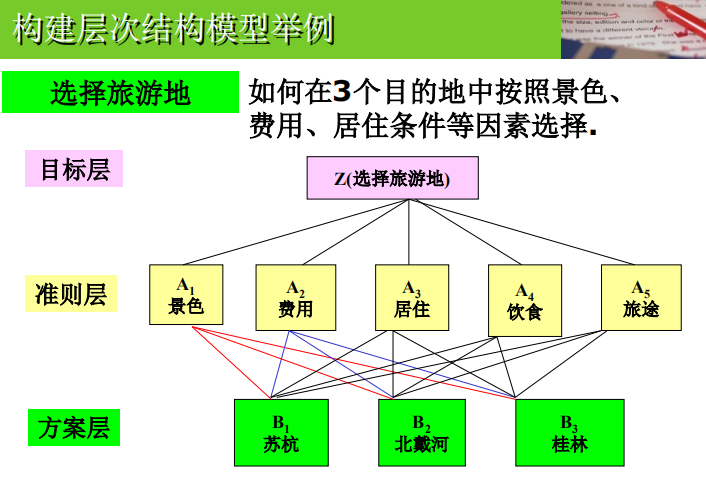## 构造成对比较矩阵

1. 不把所有因素放在一起比较，而是两两进行比较
2. 采用相对尺度,以尽可能减少性质不同的诸因素相互比较的困难，从而提高准确度。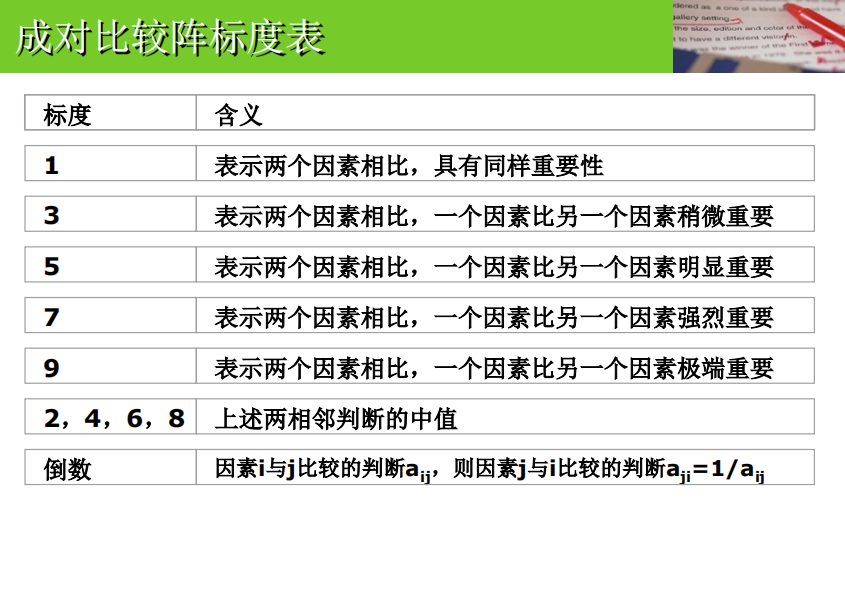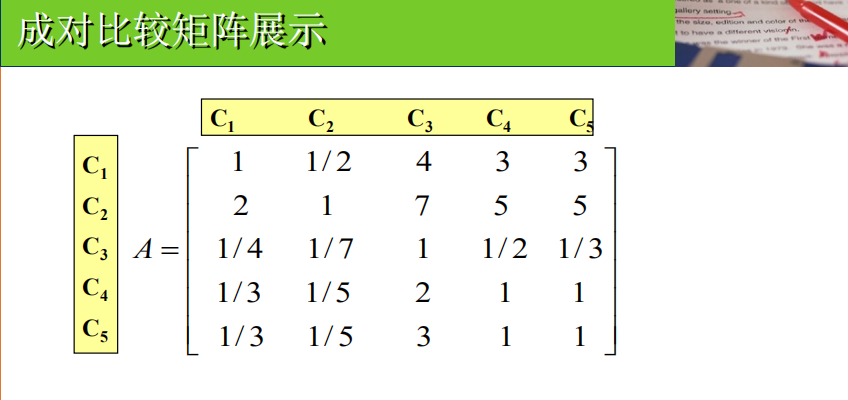## 层次单排序及其一致性检验

• 层次单排序：对于上一层某因素而言，本层次各因素的重要性的排序
• 一致性检验：按理来说应该有${a_{ik}}={a_{ij}}*{a_{jk}}$，但实际上通常不相等，只要在一定范围内即可，即允许不一致，但要确定不一致的允许范围。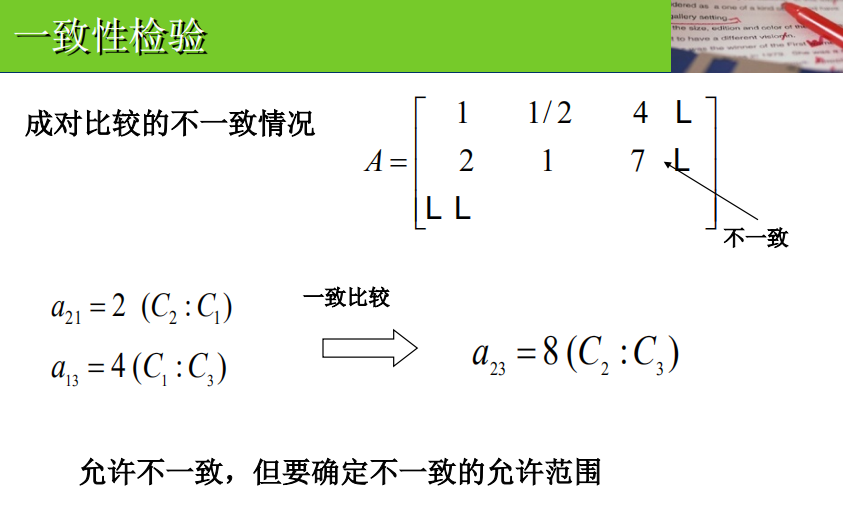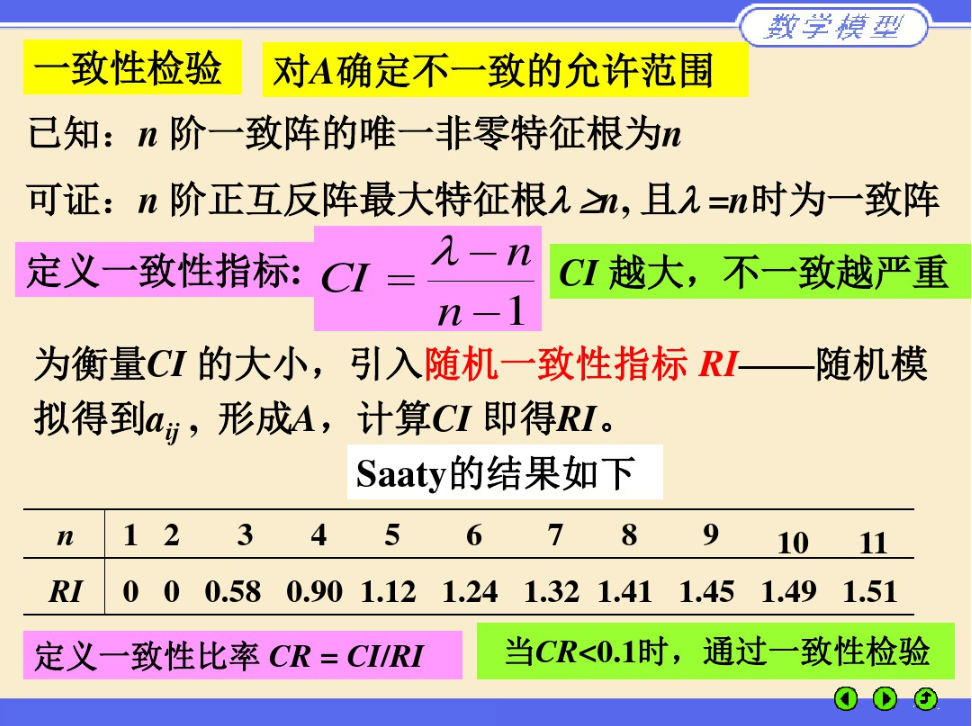## 层次总排序及其一致性检验

• 层次总排序：某层所有因素对于总目标相对重要性的排序权值过程

# 代码——matlab

disp('请输入判断矩阵A(n阶)');
A=input('A=');
[n,n]=size(A);
x=ones(n,100);
y=ones(n,100);
m=zeros(1,100);
m(1)=max(x(:,1));
y(:,1)=x(:,1);
x(:,2)=A*y(:,1);
m(2)=max(x(:,2));
y(:,2)=x(:,2)/m(2);
p=0.0001;i=2;k=abs(m(2)-m(1));
while  k>p
i=i+1;
x(:,i)=A*y(:,i-1);
m(i)=max(x(:,i));
y(:,i)=x(:,i)/m(i);
k=abs(m(i)-m(i-1));
end
a=sum(y(:,i));
w=y(:,i)/a;
t=m(i);
disp(w);
%以下是一致性检验
CI=(t-n)/(n-1);RI=[0 0 0.52 0.89 1.12 1.26 1.36 1.41 1.46 1.49 1.52 1.54 1.56 1.58 1.59];
CR=CI/RI(n);
if CR<0.10
disp('此矩阵的一致性可以接受!');
disp('CI=');disp(CI);
disp('CR=');disp(CR);
end


08-093万+

#### 数学建模四大模型总结12-16486

#### 评价类模型——层次分析法

08-266195

#### 数学建模专栏 | 第八篇：MATLAB评价型模型求解方法

07-281240

#### 数学建模之层次分析法及其应用

07-042万+

#### 数学建模————统计问题之评价（三）

09-121695

#### 数学建模 评价类问题， 层次分析法（AHP）

04-07773

#### 数学建模笔记一，层次分析法（评价类问题）

08-267293

#### 数模（1）——层次分析法

01-301万+

#### Matlab 数学建模方法（八）：评价型模型©️2020 CSDN 皮肤主题: 游动-白 设计师: 上身试试点击重新获取扫码支付1.余额是钱包充值的虚拟货币，按照1:1的比例进行支付金额的抵扣。
2.余额无法直接购买下载，可以购买VIP、C币套餐、付费专栏及课程。余额充值